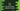# How to find the sum of two complex numbers in C++## How to find the sum of two complex numbers in C++:

Complex numbers contains one real part and imaginary part. These numbers are like x + iy where x is the real part and y is the imaginary part. To find the sum of two complex numbers, the real parts are added and the imaginary parts are added. The final number is also a complex number with its real and imaginary parts as we got in the addition. In this post, we will learn how to write one C++ program to find the sum of two complex numbers.

### C++ program to find the sum of two complex numbers:

Let’s look at the below program:

``````#include <iostream>

using namespace std;

int main()
{
int realOne, imgOne, realTwo, imgTwo;

cout << "Enter the real and imaginary parts of the first number :" << endl;
cin >> realOne >> imgOne;

cout << "Enter the real and imaginary parts of the second number :" << endl;
cin >> realTwo >> imgTwo;

int realSum = realOne + realTwo;
int imgSum = imgOne + imgTwo;
string divider = "+";

if (imgSum > 0)
{
cout << "Sum : " << realSum << divider << imgSum << "i" << endl;
}else{
cout << "Sum : " << realSum << imgSum << "i" << endl;
}
}``````

### Explanation:

• realOne, imgOne, realTwo, imgTwo are four integer values to hold the real and imaginary parts of the two numbers.
• Using cout and cin, we are reading the real and imaginary parts of the first and the second numbers.
• Variable realSum and imgSum are used to hold the real and imaginary values of the sum.
• Finally, we are printing the complex number in x+yi format.

### Sample Output:

If you run this program, it will print outputs as like below:

``````Enter the real and imaginary parts of the first number :
10 20
Enter the real and imaginary parts of the second number :
-20 -30
Sum : -10-10i

Enter the real and imaginary parts of the first number :
10 10
Enter the real and imaginary parts of the second number :
20 20
Sum : 30+30i``````

### Method 2: Using a class to hold the real and imaginary parts of the complex number:

We can also use one class to hold the real and imaginary parts of the complex numbers instead of using separate variables for each.

The class will hold two public integer variables to hold the real and imaginary parts. For example:

``````#include <iostream>

using namespace std;

class ComplexNumber{
public:
int real, imaginary;
};

int main()
{
ComplexNumber first, second, sum;

cout << "Enter the real and imaginary parts of the first number :" << endl;
cin >> first.real >> first.imaginary;

cout << "Enter the real and imaginary parts of the second number :" << endl;
cin >> second.real >> second.imaginary;

sum.real = first.real + second.real;
sum.imaginary = first.imaginary + second.imaginary;
string divider = "+";

if (sum.imaginary > 0)
{
cout << "Sum : " << sum.real << divider << sum.imaginary << "i" << endl;
}else{
cout << "Sum : " << sum.real << sum.imaginary << "i" << endl;
}
}``````
• This is similar to the above example. But we have used one new class Complex. It has two public int variables real and imaginary.
• The variables first, second and sum are of type ComplexNumber.
• Last part of this program is same as the above one.

If you run this program, it will print output as like the above one.